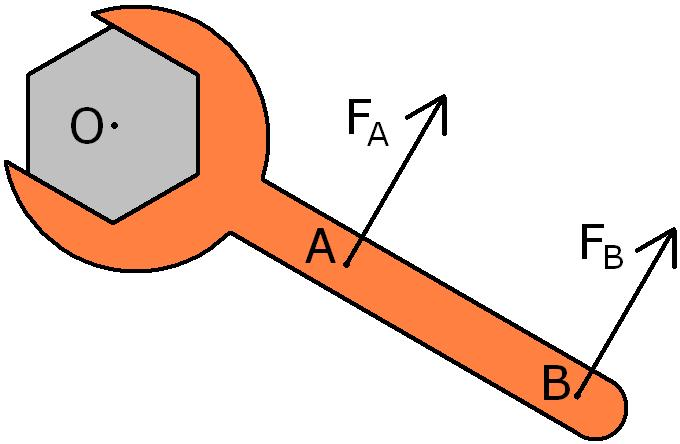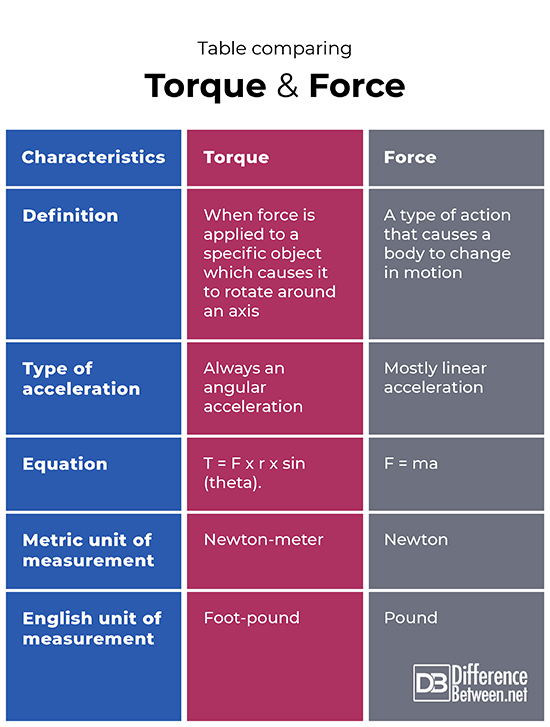#Difference Between Similar Terms and Objects

# Difference Between Torque and Force

Torque is a type of force that is applied to an object which results in the object rotating around an axis. Force is an action that causes a change in the motion of an object.## What is Torque?

### Definition:

Torque is the scientific term that is used to describe the specific effect of a force being applied to an object in which the force causes the object to rotate around an axis. Torque also concerns acceleration that is always at an angle. It is a vector but the direction varies according to the direction of the force that is being applied. The amount of force needed to bring about movement with torque decreases as distance increases from the point of rotation.

### How it is measured:

There is an equation that can be used for calculating torque, namely, T = F x r x sin (theta). In this equation F is a linear force; r is the distance between the force and the axis of rotation, while theta is the angle between both the axis of rotation and the application of the linear force. The unit of measurement used for torque in the metric system is the Newton-meter (symbol N-m). The English unit that is used for the measurement of torque is the foot-pound. The units include distance because torque is related to distance as well as to the force that is being applied to the object.

### Examples:

There are multiple examples of torque from everyday life. For instance, turning a key in a lock or even turning a door knob to open a door are all examples of torque. Levers work based on the principle of torque as well, and anybody who has had to loosen a lug nut understands the motion involved with torque.

### Application:

Knowing about torque is important in engineering and the design of levers and even in the development and design of internal combustion engines. Knowing torque is also useful when it comes to calculating how quickly a car can accelerate.## What is Force?

### Definition:

Force is some type of action that occurs, which either keeps a body in motion or changes the motion of the body in some fashion. The concept is based on Newton’s three laws of motion. Force is a quantity that is a vector since it has not only size but also a specific direction associated with it, and it involves, most often, the linear acceleration of objects. There are many different types of forces including natural forces such as gravity, electromagnetic force, tension and friction.

### How it is measured:

The unit of measurement that is used for force is the Newton (symbol N). A Newton is equivalent to the amount of force that is required in order to set in motion and move an object weighing one kilogram a distance of one meter per second per second. The English system of units uses the pound as the unit of measure of force. In this case, one pound of force is seen as equivalent to the acceleration at 32.17 feet per second of a one pound object. Force (F) can often be calculated as mass (m) times acceleration (a), so the equation is F = ma. Based on this equation, the acceleration of an object will be related to the force and mass of the object. This means that more force would be needed to move an object that is of a higher mass than an object that is of a lower mass.

### Examples:

There are many examples of forces from everyday life in which a force is applied to an object. For example, kicking a ball or pushing a box all involve a force being applied to an object of a particular mass. There are also several types of natural forces that have been defined and categorized, such as gravitational force and electromagnetic force.

### Application:

Understanding forces is crucial to the design of many man-made structures. Knowledge of natural forces such as gravity and electromagnetism are also important in many fields of science.

## Difference between Torque and Force?

### Definition

Torque is when a force is applied to a particular object that results in the object moving by rotating around an axis. A force is some type of action that results in a body changing in motion or altering the path of motion.

### Type of acceleration

The type of acceleration involved with torque is always angular, while the type of acceleration of force is mostly linear.

### Equation

The mathematical equation that is often used to calculate torque is T = F x r x sin (theta). The mathematical equation that is often used to determine force is F = ma.

### Metric unit of measurement

The Newton-meter is the metric unit of measurement that is used when calculating torque. The Newton is the metric unit used when measuring force.

### English unit of measurement

The English unit of measurement which is used for torque is the foot-pound. The English unit of measurement which is used for force is the pound.

### Table comparing Torque and Force## Summary of Torque Vs. Force

• Torque and force measurements are both important to know when it comes to engineering.
• Torque always involves acceleration at an angle and it involves distance as well as force.
• Force is the action that causes some type of change in motion of an object.
• There are many different types of forces that can be calculated or need to be taken into account, including natural forces such as gravity and electromagnetism.

### Search DifferenceBetween.net :

Custom Search

Help us improve. Rate this post!Loading...Email This Post : If you like this article or our site. Please spread the word. Share it with your friends/family.

Please note: comment moderation is enabled and may delay your comment. There is no need to resubmit your comment.

## References :